+关注继续查看

@[toc]

# 1. 背景

## 计算机和编程语言

//例：hello world!
#include<stdio.h>
int main()
{
printf("Hello World!\n");//输出“Hello World!（换行）”引号内的部分
return 0;
}


（算法：我们想让计算机做计算，先要写出计算的步骤，然后用编程语言写出来。计算机做的所有事情都是计算；计算的步骤就是算法。）

## 计算机的思维方式

1) 枚举法

2) 二分法

1) 解释：借助一个程序，那个程序可以试图理解你的程序，然后按照你的要求执行
2) 编译：借助一个程序把你的程序翻译成机器语言写的程序，然后计算机就可以直接执行了。

## 为什么编程入门从C学起？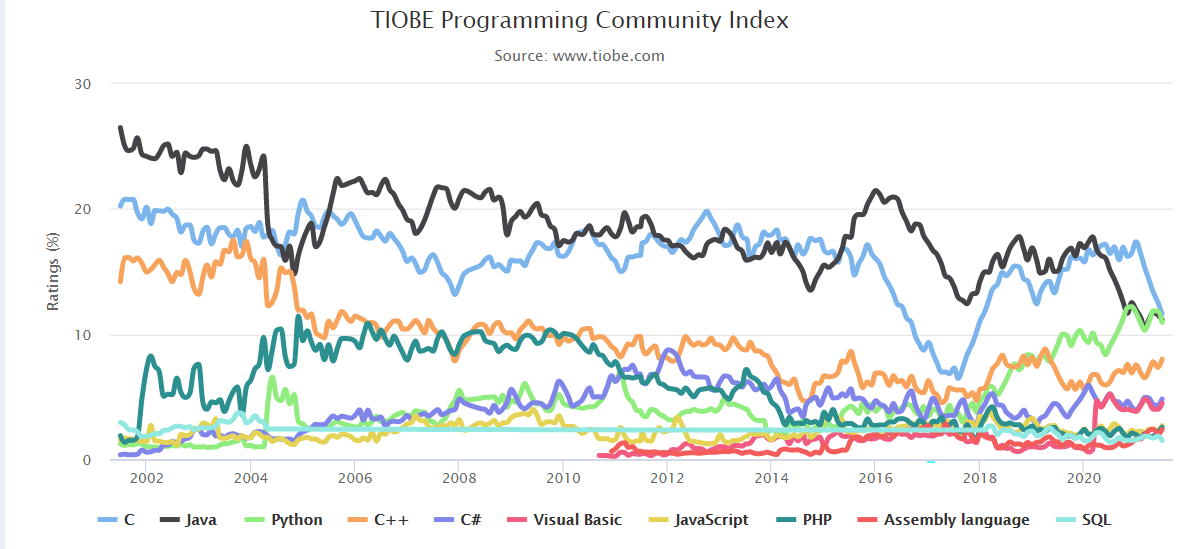1) 在很多场合，C语言是唯一的选择
2) 现代编程语言在语法上差异很小，基本都和C接近
3) 语言的能力、适用领域主要是由 传统 决定的。

## C的简单历史

1969诞生。
FORTRAN→BCPL→B语言→C语言

*1967年，剑桥大学的 Martin Richards 对CPL语言进行了简化，于是产生了BCPL（Basic Combined Pogramming Language)语言。
1970年，美国贝尔实验室的 Ken Thompson。以BCPL语言为基础，设计出很简单且很接近硬件的B语言（取BCPL的首字母）。并且他用B语言写了第一个UNIX操作系统。

C语言还受到了PL/I（和底层系统很接近，可以拿来做操作系统等）的影响，还和 PDP-II的机器语言有很大关系
1973/3，第三版的Unix上出现了C语言的编译器
1973/11，第四版的Unix完全用C语言重新写的。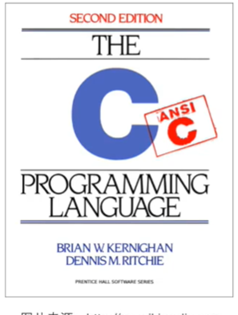1989年，ANSI发布了一个标准：ANSI C
1990年，ISO接受了ANSI的标准：C89
C的标准在1995年和1999年两次更新：C95和C99。现在的编译器都基于C99了，因此本课程也是。

## 编程软件用什么

C语言的用途：写操作系统、嵌入式系统、驱动程序（用来驱动U盘）、底层驱动、图形引擎、图像处理、声音效果（直接给我们提供数据的代码不是用C语言写的）一些底层的东西。
C语言是一种工业语言，由基础人员使用。所以：
**开发效率>>学习过程

C语言需要被编译才能运行，所以需要：编辑器和编译器
（或者IDE，Integrated Development Environment集成开发环境，一个顶俩）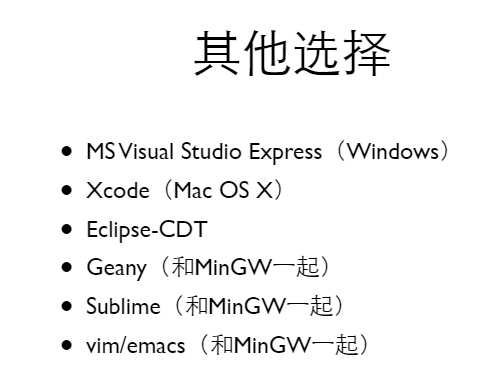# 2. 入门：从Hello World开始

## 第一个C程序

#include<stdio.h>
int main()
{
printf("Hello World!\n");
return 0;
}

**上端显示星号，说明没有保存。Ctrl + S 保存并且记得选择.c格式。

## 详解第一个C程序

#include<stdio.h>
int main()
{

return 0;
}

printf()会把" "内的内容（字符串）原封不动地输出，\n表示换行。

## 简单计算

printf("%d",23+43);

%d是占位符，说明后面会有一个整数输出到这个位置上。

printf("23+43=%d",23+43);

++
--
×*
÷/
%取余
( )( )括号

# 3. 变量

## 变量定义

1) 有办法输入数字；
2) 有地方放输入的数字；
3) 输入的数字参与计算。

    int price=0;//定义了整形变量price，类型是int，初始值=0
printf("请输入金额（元）：");
int change=100-price;
printf("找您%d元。\n",change);

<类型名称> <变量名称>

（敲下回车之前，程序不会读到任何东西。）

## 变量赋值与初始化

    int price=0;//其中=是赋值运算符，把右边的值赋给左边的变量。

    int a=0,b=1;

C语言是有类型的语言，所有变量在使用前必须先定义或声明；所有变量必须有确定的数据类型（表示在变量中可以存放什么样的数据），变量中也只能存放指定类型的数据，程序运行过程中也不能改变变量的类型。
int charge=100-price;

//C语言
int price=0;
printf("请输入金额（元）：");
scanf("%d",&price);
int change=100-price;
printf("找您%d元。\n",change);
//传统的ANSI C只能在开头的地方定义变量
int price=0;
int change=0;

printf("请输入金额（元）：");
scanf("%d",&price);
change=100-price;
printf("找您%d元。\n",change);

## 变量输入

scanf()函数，读到的结果赋值给后面的变量
（注意变量前的&）

## 常量vs变量

    const int amount=100;

（一般const的变量全大写）
scanf的空格有讲究，以后再说。输入时如果要两个数，在中间、最后敲空格或回车，计算机读到两个数字时停止

## 浮点数

C语言中两个整数做运算得到的结果也只能是个整数（去掉小数部分）。而10和10.0在c中是完全不同的两个数字，10.0是浮点数（浮点数指小数点时可以浮动的，是计算机中表示分数和无理数的一种方式。人们用浮点数来称呼有小数点的数）（其实还有定点数。但是在c语言中无）

（如：

int a,b;
printf("a/b*3");//改进为a/b*3.0

(计算机里会有这种纯粹的整数，是因为运算快、占地小。而且日常生活中大多也是整数运算。)

## 表达式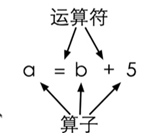int hour1,min1;
int hour2,min2;

scanf("%d %d",&hour1,&min1);
scanf("%d %d",&hour2,&min2);

int t1=hour1*60+min1;//把小时转化为分钟单位
int t2=hour2*60+min2;

int t=t2-t1;
printf("时间差是%d小时%d分。“,t/60,t%60);//t/60是小时部分；t%60取余，是分钟部分

## 运算符优先级

（double输入的时候用%lf，输出的时候用%f就行）

1+单目不变自右向左a*+b
1-单目取负自右向左a*-b
2*自左向右a*b
2/自左向右a/b
2%取余自左向右a%b
3+自左向右a+b
3-自左向右a-b
4=赋值自右向左a=b
（a+b,a-b是双目；+a,-a是单目，相当于正负号）

a=6的结果就是a被赋予的值，也就是6
a=b=6：自右向左，a=(b=6)。

    result=a=b=3+c;
result=(result=result*2)*6*(result=3+result);

（C语言没有幂次，所以只能一个个乘。）

## 交换变量

a=b;
b=a;

c=a;
a=b;
b=c;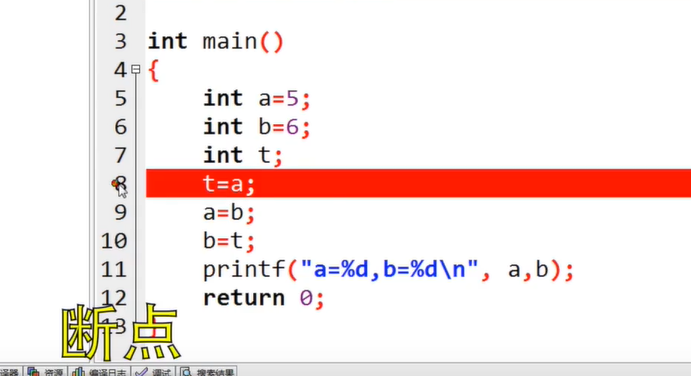DEV C++中，在左边数字那里点击一下，然后程序运行时就会在这一行停止。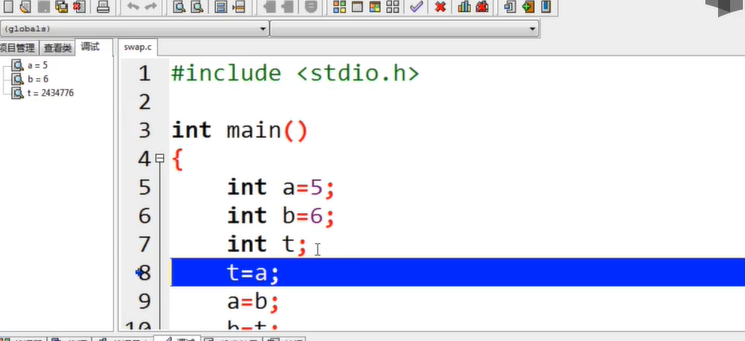C语言：使用Dev C++断点调试

## 复合赋值

a+=5表示a=a+5(注意两个运算符之间无空格)

a*=b+5表示a=a*(b+5),如果想先算a=a*b记得加括号）
INC递增、DEC递减运算符：++--：单目运算符，只能有一个算子，这个算子还只能是变量（如a++后缀形式，++a前缀形式）让该变量+1/-1；
a++运算式的值是a+1以前的值；++a运算式的值是a+1以后的值。无论哪个，a的值都+1了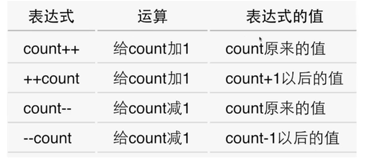++--可以单独使用，但不要把它组合进表达式，复杂。

# 4. 判断（选择结构）

if条件判断

    if(条件){
要执行的语句，只有条件成立时才会执行
}

## 判断的条件

==判断相等
!=不相等

printf("%d\n",5>3);
printf("%d\n",5==3);

(编程语言都是可以人为验证的)
int r=a>0;先判断a是否>0,再把0/1的值赋给r
==!=优先级比其他的低

5>3==6>41==1成立
6>5>41>4不成立

## 找零计算器

flowchat
st=>start: 开始
i1=>inputoutput: 输入购买的金额
i2=>inputoutput: 输入支付的票面
o1=>operation: 计算找零
c=>condition: 判断余额是否充足
o2=>inputoutput: 打印找零
o3=>inputoutput: 告知用户余额不足以购买
e=>end: 结束

st->i1->i2->o1->c
c(yes)->o2->e
c(no)->o3->e
    //初始化
int price=0;
int bill=0;
//读入金额和票面
printf("请输入金额：");
scanf("%d",&price);
printf("请输入票面：");
scanf("%d",&bill);
//计算找零

//是（单行）注释，把程序分成了三个部分：初始化，读入金额和票面，计算并打印找零；
c99可以，ASCII不支持
comment对程序的功能没有任何影响，但是往往能使程序更容易被人类读者理解。
/* */中间可以有多行注释。

## 否则

else{ }if的补集

    int a,b;
printf("请输入两个整数：");
scanf("%d %d",&a,&b);
int max=0;
if(a>b)max=a;
else max=b;
printf("大的那个是%d\n",max);

    int a,b;
printf("请输入两个整数：");
scanf("%d %d",&a,&b);
int max=b;
if(a>b)max=a;
printf("大的那个是%d\n",max);

## 没有大括号的if语句

if语句可以不加大括号

if（a>b）
max=a;

If这行结束并没有语句结束的标志;而后面的赋值语句写在下一行且缩进了，结束时有“；”。这表明这条赋值语句是if语句的一部分，if语句拥有和控制这条赋值语句，决定他是否要被执行。

## 嵌套的if-else

if(a>b)
{
if(a>c)max=a;
else max=c;
}
else
{
if(b>c)max=b;
else max=c;
}


## 级联的if-else if

flowchat
st=>start: 开始
i=>inputoutput: 输入
o=>inputoutput: 输出
c1=>condition: 是否满足条件1
c2=>condition: 是否满足条件2
o1=>inputoutput: 输出条件1
o2=>inputoutput: 输出条件2
o3=>inputoutput: 输出条件3
en=>end: 结束
st->i->c1
c1(yes)->o1->en
c1(no)->c2
c2(yes)->o2->en
c2(no)->o3->en

else
{
if()
else
}

if(x<0)f=-1;
else if(x==0)f=0;
else f=1;
printf("%d",f);
if(x<0)printf("%d",-1);
else if(x==0)printf("%d",0);
else printf("%d",1);

## if-else的常见错误

1. 忘记写大括号的话，条件成立时只执行后面一句
2. If后面无分号
3. ==和=：if只要求括号里是0或非0
4. 使人困惑的else

3.2.4 多路分支

switch(print){
case 1:
printf("1");
break;
case 2:
printf("2");
break;
case 3:
printf("3");
break;
default:
printf("0");
}

Switch是先跳到对应的case处，然后一条条向下执行直到遇到break跳出switch

int i=1;
switch ( i%3 ) {
case 0: printf("zero");
case 1: printf("one");
case 2: printf("two");
}

# 5. 循环（循环结构）

if(n!=0)
{
n/=10;i++;
}
if(n!=0)
{
n/=10;i++;
}

……不过这样也是无限循环。

（尝试的时候不要拿太大的数去试，计算机里的整数是有范围的。）

## while 循环

1. 用户输入x；
2. 初始化n为0；
3. X/=10，去掉个位；
4. cnt++;
5. 如果x>0，回到3；
6. 否则cnt就是结果。

do-while循环：进入循环的时候不做检查，而是执行完一轮循环体的代码之后再来检查循环条件是否满足，满足的话继续，不满足的话结束循环
(while();最后一定要有分号！！！)
while和do while很像，区别在于do while在循环体执行结束时才来判断条件。也就是说无论如何，循环都会执行至少一遍。

## do-while循环

if和while的区别在于，if判断只一次，不管结果如何都结束了。
While一定要有结束循环的条件！！否则会一直循环，超时
While可以翻译为当。循环有可能一次都没有被执行。条件成立是循环成立的条件。

（此题求位数，特殊数据可以是个位数、10、0、负数）

## 循环计算

• While可以用do while吗？
• 为什么计数从0开始，可以从1开始吗？
• 为什么while判断条件是x>1?
• 循环最后输出的是多少？
#include<stdio.h>
int main()
{
int x,ret=0;
scanf("%d",&x);
int t=x;
while(x>1){
x/=2;
ret++;
}
printf("log2 of %d is %d.",t,ret);
return 0;
}

1、 当x=1的时候，结果是0.也就是说我们希望当x=1时不要进入这个循环。
2、 计数ret是我们希望进入这个循环是最小的数。

## 猜数游戏

1) 因为要不断重复去猜，所以我们要用到循环
2) 实际写出程序之前，我们可以先用文字描述出程序的思路。
3) 核心重点是循环的条件。
4) 人们往往会考虑循环终止的条件。

flowchat
st=>start: 开始
i=>inputoutput: 用户输入猜的数
ocnt=>operation: count++
o=>operation: 计算机随机想一个数，记在number里
c=>condition: 判断a是否等于number
e=>end: 结束
o2=>inputoutput: 告诉用户大了还是小了
oe=>operation: 输出cnt猜的次数

st->o->i->ocnt->c
c(yes)->oe->e
c(no)->o2(right)->i

//先加入两个头文件#include<stdlib.h>和#include<time.h>
#include<stdio.h>
#include<stdlib.h>
#include<time.h>
int main()
{
srand(time(0));//Main里加上srand(time(0));  先不用管什么意思
int a=rand();//如果想要a是100以内的整数：用取余即可（a%=100）
int number=rand()%100+1;//这样召唤出来的数范围是1~100
//不管怎样用户都要进入这个循环，输入至少一个数；所以应该用do-while循环。
}

## 算平均数

num:我们需要读到的那一个数。
sum(总和)：每读到一个数把他加到sum里就完事了

flowchat
st=>start: sum=0,cnt=0
i1=>inputoutput: 读num
c=>condition: num!=-1?
o=>operation: sum+=num, cnt++
op=>inputoutput: 计算和打印结果
e=>end: end
st->i1->c
c(yes)->o->i1
c(no,left)->op->e

## for循环

for循环像一个计数器。达到一个数之前一直进行循环，在过程中i++i--
for(int i=0;i<n;i++)n次循环

still, 可以尝试细节。

## 循环控制

break:结束循环
continue:可以跳过此循环剩下的部分，进入下一轮循环## 嵌套的循环

int x;//再scan x
int isprime=1;
for(int i=2;i<x;i++){
if(x%i==0){
isprime=0;
break;
}
}//再根据isprime是不是1判断x是不是素数

/t可以对齐，具体以后再讲

## 从嵌套的循环中跳出

for(one=1;one<x*10;one++)
{
for(two=1;two<x*10/2;two++)
{
for(five=1;five<x*10/5;five++)//break跳出的是这层循环，而没有跳出上面的两层循环
{
if(one+two*2+five*5==x*10)
{
printf("可以用%d个一角加%d个两角加%d个五角得到%d元\n",one,two,five,x);
break;
}
}
}
}

if内写 exit=1;

//接力break
int x,one,two,five,x;
int exit=0;
scanf("%d",&x);
for(one=1;one<x*10;one++)
{
for(two=1;two<x*10/2;two++)
{
for(five=1;five<x*10/5;five++)
{
if(one+two*2+five*5==x*10)
{
printf("可以用%d个一角加%d个两角加%d个五角得到%d元\n",one,two,five,x);
exit=1;
break;
}
}
if(exit==1)break;
}
if(exit==1)break;
}

//goto
int x,one,two,five,x;
int exit=0;
scanf("%d",&x);
for(one=1;one<x*10;one++)
{
for(two=1;two<x*10/2;two++)
{
for(five=1;five<x*10/5;five++)
{
if(one+two*2+five*5==x*10)
{
printf("可以用%d个一角加%d个两角加%d个五角得到%d元\n",one,two,five,x);
goto out;
}
}
}
}
out:
return 0;

（除了这种多重break的结构建议使用goto，别的地方不建议。）

# 补充：PTA 例题

## 前n项和

#include<stdio.h>
int main()
{
int n;
double sum=0.0;
scanf("%d",&n);
for(int i=1;i<=n;i++)
{
sum+=1.0/i;
}
printf("f(%d)=%f",n,sum);
return 0;
}

sum+=sign*1.0/i;
sign=-sign;

## 整数分解

1. 先求x的逆序数t
2. 逆序逐位输出t

123456 / 100000 = 1
123456%100000 = 23456
23456 / 10000 = 2
……

## 求最大公约数

循环t++
if(u/t==0&&v/t==0)gcd=t;//不断刷新gcd
if(t==u||t==v)break;//并输出gcd;

1. 如果b=0，计算结束，a就是最大公约数；
2. 否则，让a=b，b=a%b；
3. 回到第一步

（可以使用之前提到过的变量表格法来人工运行）

## 求符合给定条件的整数集

234 235 243 245 253 254
324 325 342 345 352 354
423 425 432 435 452 453
523 524 532 534 542 543

int i,a,j,k,cnt=0;
scanf("%d",&a);
i=a;
//思路：i,j,k都从a开始，从小到大逐渐增加到a+3，三者不能相等

while(i<=a+3){
j=a;
while(j<=a+3){
k=a;
while(k<=a+3){
if(i!=j&&j!=k&&i!=k){
printf("%d%d%d",i,j,k);
cnt++;
if(cnt==6){
printf("\n");
cnt=0;
}
else printf(" ");
}
k++;
}
j++;
}
i++;
}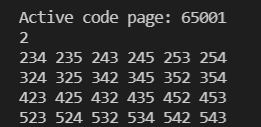153
370
371

## 打印乘法口诀表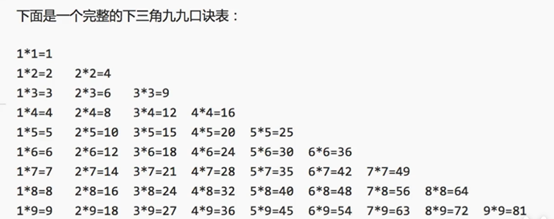a*b，a、b两重循环。

## 猜数游戏

//也不难，1.考的是语文的阅读理解；2.你是否有足够的耐心。（文中条件太多）

## n项求和

2/1+3/2+5/3+8/5+……的前n项之和（从第二项开始，每一项的分子是前一项的分子和分母之和，分母是前一项的分子）

dividend=2;//分母
divisor=1;//分子
for(i=1;i<=n;i++){
sum+=dividend/divisor;
t=dividend;
dividend+=divisor;
divisor=t;
}
printf("%.2f/n",sum);

(inf是越界，即无穷；nan是无效数字)

## 约分最简分式

1. scanf("%d/%d")这样处理输入
2. 辗转相除法寻找最大公约数；

# 6. 数据类型

C语言是有类型的语言。C语言的变量，必须：
1.在使用前定义;
2.确定类型.

C语言之后语言朝着两个方向发展：

1. C++和Java更强调类型，对类型的检查更加严格
2. JavaScript、Python、PHP不着重类型，甚至不需要事先定义。

C语言需要类型，但是对类型的安全检查并不足够：
（以下斜体的是c99类型）

1. 类型名称：int,long,double……
2. 输入输出时的格式化（占位符）：%d,%ld,%lf……
3. 所表达的数的范围：char<short<int<float<double
4. 内存中占据的大小：1~16个字节

Sizeof()是一个运算符，给出某个类型或变量在内存中所占据的字节数。如：
sizeof(int)
sizeof(i)
(1个字节是8个比特，所以int4个字节32个比特)
sizeof是一个静态运算符，在编译时其结果就已经决定了

## 整数类型

sizeof(char)=1;//char1字节（8比特）
sizeof(short)=2;//short2字节
sizeof(int)=4;//由编译器决定，通常代表“1个字”
sizeof(long)=4;//由编译器决定，通常代表“1个字”
sizeof(long long)=8;//long long 8字节## 整数的内部表达

1. 像十进制一样，有一种特殊的标志（类似符号）来表示负数（缺陷：计算机做加减法的时候，要像判断十进制的负号一样，我们需要一个东西去控制加号还是减号。不过每次计算机都要特别地去判断这个符号的正负，这样就会比较复杂。）
2. 从0000 0000到1111 11111，取中间的数为0，如1000 0000表示0，比他小的是负数，比他大的是正数，各一半（缺陷：所有数都要和这个数做减法来计算其值，会比较复杂）
3. 补码

（1111 1111被当作纯二进制看待时是255，被当做补码看待时是-1）

## 整数的范围：如何推算整数类型所能表达的数的范围，越界了会怎样？

0000 0001 ~ 0111 1111→1~127（纯二进制数）
1000 0000 ~ 1111 1111→-128 ~ -1（用补码表示的数）

char c=255;
int i=255;
printf("%d%d",c,i);

char1字节-128~127
short2字节-2^15^~2^15^-1
int取决于编译器-2^31^~2^31^-1
long4字节-2^31^~2^31^-1
long long8字节-2^63^~2^63^-1

unsigned char c=255;

unsigned使得这个类型在正整数表达部分范围扩大一倍，但是不能表达负数了。

Similarly,想表达自己是个long,后面加l
unsigned的初衷不是为了扩展数能表达的范围，而是为了做纯二进制运算，主要是为了移位。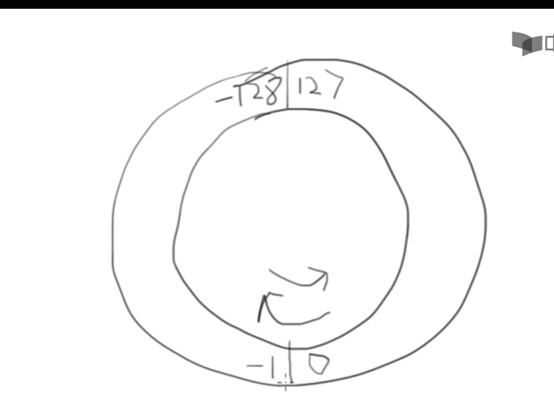char c=127;
c++;

Similarly，-128-1变成了127。
unsigned（无符号）则是直接加减。

unsigned char c=255;
c++;

c=0;
c--;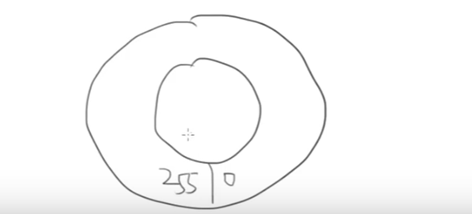int a=0,b=1;
while(++a>0);
printf("int数据类型的最大数是：%d\n",a-1);
while((a/=10)!=0)b++;
printf("int数据类型最大的数的数位是:%d",b);

## 整数的格式化：如何格式化地输入输出整数，如何处理8进制/16进制

%d：int
%u: unsigned
%ld: long long
%lu: unsigned long long

8进制（octal）：一个以0开始的数字字面量

 char c=012;
int i=0x12;
printf("c=%d,i=%d\n",c,i);

（计算机内部同样还是二进制的形式）

(但是这样输出的结果前面是没有0和0x的。所以可以写printf(“c=0%o,i=0x%x\n”,c,i);
8进制和16进制只是如何把数字表达为字符串，与内部如何表达数字无关。
scanf中也可以用%o，表示读进来的数我们把其当做8进制来读。
16进制很适合表达二进制数据，因为4位二进制正好是一个16进制位（0001 0010→1 2）

## 整数类型的选择

char,short,int,long,long long

C语言这些又多又复杂的原因：1.C语言的严谨性，为了准确表达内存，做底层程序的需要
2.C语言要和硬件打交道，要准确表达计算机里的东西（内存、寄存器、接口……）

1) 现在CPU字长（CPU和内存之间的通道。如果char可能会从32位中先挑出来8个，可能会花费时间）普遍是32位/64位，一次内存读写、一次计算都是int，选择更短的类型不会更快，甚至可能会更慢
2) 现代编译器一般会设计内存对齐，（比如这个东西虽然占据8bit，在内存中仍然占据了一个int。这个事等到结构再讲）所以更短的类型实际在内存中也可能占据一个int的大小。除非在做顶层的硬件，告诉你硬件多大你就要用多大去运算

## 浮点类型

float，double
32位，64位
10^38^,10^308^
7位有效数字，15

float还能表达0，+-inf（无穷），nan（非有效数字）
%e/%E:科学计数法
1234.56789输出得到：1.234567e+03
-5.67E+16，科学计数法可选的有：+-号，e或E，小数点，E后面的符号+-
Double ff=1E-10；

%.3f,-0.0045→-0.004
%.30f→后面输出30位，-0.0049→-0.00489999……（计算机最终只能用离散的数字来表示数字，这就是浮点数的误差。Double更准确，但可能依然不精确）
%.3f，-0.00049→-0.000

## 浮点数的范围和精度

Inf无穷，nan输出不存在的浮点数

0.0/0.0→nan
(如果是整数，12/0编译不过)

float a,b,c;
a=1.345f;
b=1.123f;
c=a+b;
if(c==2.468)printf("相等");
else printf("不相等，c=%.10f，或%f",c,c);

f1==f2不一定成功，应该写fabs(f1-f2)<1e-12

Android计算器低级错误？都是二进制惹的祸！## 字符类型：char是整数也是字符

char是整数，也是字符。（character）

''也是字符

scanf("%d%c",&i,&c);
scanf("%d %c",&i,&c); 

int i='Z'-'A';//两个字符相减，得到它们在表中的距离

'a'-'A'可以得到小写字母与大写字母之间的距离，所以'a'+('A'-'a')可以转小写为大写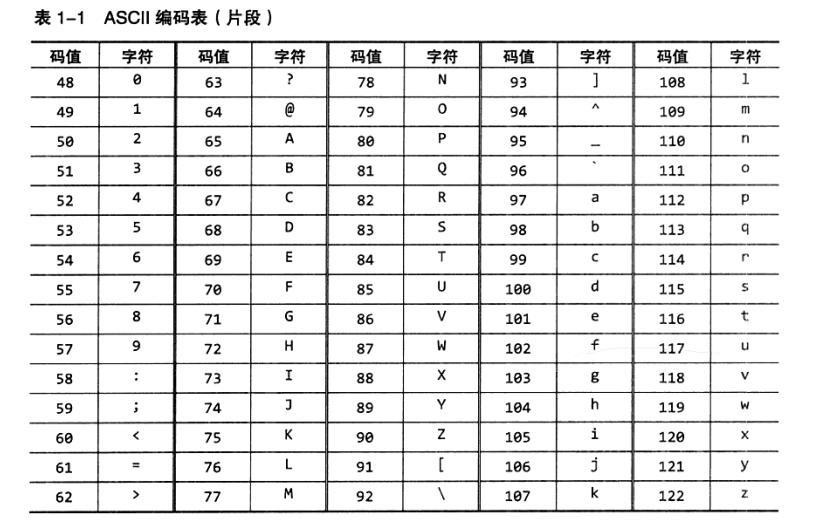## 逃逸字符

printf("请分别输入身高的英尺和英寸，""如输入\"5 7\"表示5英尺7英寸：");

\b回退一格\"双引号
\t到下一个表格位\'单引号
\n换行\反斜杠本身
\r回车

（作业时就会有最后结尾多个空格，使用/b来删掉的情况，但其实应该说是覆盖了吧，总之这样运行的时候是不正确的，还是要想办法控制输出的空格等的格式正确）

printf("123\b\n456\n");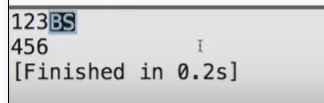123
456

\b\n都是控制字符
BS是回到上一格，没输出东西就什么结果都没有，输出了就把他盖住了

\t:到下一个表格位（在每一行中有一些固定的位置，\t代表输出的固定的位置）

printf("123\t456\n");
printf("12\t456\n");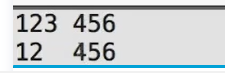\n换行\r回车，源自打字机的动作

## 类型转换

char->short->int->long->long long
int->float->double

(int)10.2;
(short)32;

int i=32768;
short s=(short)i;
printf("%d\n",i);

i输出仍然是32768。强制类型转换不会改变这个变量自身的类型或值。

double a=1.0;
double b=2.0;
int i=(int)a/b;

int i=(int)(a/b);
(double)(a/b);

## 逻辑类型：表示关系运算和逻辑运算结果的量

bool类型

bool b=6>5;

## 逻辑运算：对逻辑量进行与、或、非运算

!逻辑非!aa的true或false反转
&&逻辑与a&&b只有a&b都是true时结果才是true
\\ 逻辑或a\\b只有a&b都是false时结果才是false

x>4&&x<6x>=4&&x<=6

1()从左到右
2!，+，-，++，--从右到左（单目的+和-）
3*，/，%从左到右
4+，-从左到右
5<，<=，>，>=从左到右
6==，!=从左到右
7&&从左到右
8\\ 从左到右
9=，+=，-=，*=，/=，%=从右到左

a==6&&b==1如果左边a!=6就终止了。

int a=-1;
if(a>0&&a++>1){
printf("OK\n");
}printf("%d\n",a);

## 条件运算符

cnt=(cnt>20)?cnt-10:cnt+10;
//格式：条件?条件满足时的值:条件不满足时的值

if(cnt>20)cnt-=10;
else cnt+=10;

i=3+4,5+6;//编译时会提示warning。因为赋值的优先级也要高于逗号，该式子其实是
(i=3+4),(5+6);//右边编译错误,i=7

for(i=0,j=10;i<j; i++,j--)

# 7. 函数

## 初见函数

int isPrime(int i)
{
int ret=1;
int k;
for(k=2;k<i-1;k++){
if(i%k==0){
ret=0;
break;//有1~i-1间的因数就可以判断i不是素数了，break节约时间
}
}
return ret;
}

PS: 程序中出现几段几乎完全相似的代码（除了字母不同），“代码复制”是程序不良的表现。因为将来做修改、维护的时候要维护很多处。
（吓得我立刻就去改大作业了）我们用函数替换，可以把重复的代码提出。

//闭区间内所有数求和的函数
void sum(int begin,int end)
{
int i,sum=0;
for(i=begin;i<=end;i++){
sum+=i;
}
printf("%d到%d的和是%d\n",begin,end,sum);
}

int main()
{
sum(1,10);
return 0;
}

## 函数的定义和调用

（）起到了表示函数调用的重要作用，即使没有参数我们也需要（）。

## 从函数中返回

int函数会有返回值return
return停止函数的执行，并返回一个值
return;
return 一个值;

## 函数原型：用来告诉编译器这个函数长什么样

C的编译器自上而下分析你的代码，它会记住函数sum()长什么样子要几个参数，每个参数的类型如何，返回什么类型

（事先声明了函数的样子）

void sum(int begin,int end);//声明

int main()
{
//略去不表，其中用到了sum(a,b)
}

void sum(int begin,int end)//定义
{
int i,sum=0;
for(i=begin;i<=end;i++){
sum+=i;
}
printf("%d到%d的和是%d\n",begin,end,sum);
}

double max(double a,double b);

Void sum(int ,int)

## 参数传递：调用哪个函数的时候，是用表达式的值来初始化函数的参数

 void swap(int a,int b)//形参

int main()
{
int a=5;
b=6;
swap(a,b);//实参
}
void swap(int a,int b)//形参
{
int t=a;
a=b;
b=t;
}

C语言在调用函数时，永远只能传值给函数。

（见上图）

## 本地变量：定义在函数内部的变量是本地变量，参数也是本地变量

### 变量的生存期和作用域

void swap(int a,int b)//形参

int main()
{
int a=5;
b=6;
swap(a,b);//实参
}
void swap(int x,int y)//形参
{
int t=x;
x=y;
y=t;
}

1. 本地变量定义在块内。可以是函数的块内，语句的块内，如：

if (a<b)int i=10;

## 函数庶事：一些细节，main()的解释

C语言不允许函数嵌套定义。
（也最好不要写return (i);虽然意思上没变，但是会让人误会return 是个函数）
int main(void)也是个函数，所以return 0;也是有意义的。

# 8. 数组

## 初试数组

int number;//定义数组，表示数组可以放100个int
scanf(“%d”,&x);
while(x!=-1){
number[cnt]=x;//对数组中的元素赋值
cnt++;
scanf(“%d”,&x);
}

if(cnt>0){
int i;
double average=sum/cnt;
for(i=0;i<cnt;i++){
if(number[i]>average){//使用数组中的元素
printf("%d ",number[i]);//遍历数组
}
}
}

## 数组的使用：如何定义和使用数组，数组的下标和下标的范围

<类型>变量名称[元素数量];//方括号表示这是个数组

int grades;
double weight;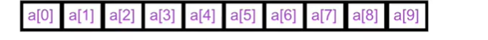（要习惯数数从0开始到n-1）

## 数组的例子：统计个数

for(int i=0;i<10;i++)count[i]=0;

for(int i=0;i<10;i++)printf(“%d\n”,count[i]);

1. 确定数组大小；
2. 定义数组；
3. 初始化数组；
4. 数组参与运算；
5. 遍历数组输出。

## 数组运算

（往函数中传数组：int sum(a[])）

int a[]={2,4,6,7,1,3,5,9}
/*直接用大括号给出数组所有元素的初始值；

C99还可以在大括号里给指定的位置赋值。

int a={=2,=3,6};

int a[]={=2,=3,6};

## 数组的大小

sizeof给出整个数组所占据的内容的大小，单位是字节。（n*4，sizeof(a)/sizeof(a)就能得到数组元素个数）

==数组变量本身不能被赋值==。如果想把一个数组的值全部交给另一个数组，必须遍历。

## 遍历数组

2.离开循环之后继续使用i作为数组元素下标。

2.不能在[]中给出数组的大小。

## 数组例子：素数

isPrime()函数：我们从2到x-1都拿去除x，循环要走很多遍，重复执行的次数很多（程序很差）。

for(int i=3;i<x;i+=2)

for(int i=3;i<=sqrt(x);i+=2)

Windows用户：打开浏览器搜索。

int isPrime(int x,int knowsPrimes[],int numberofKnownPrimes)
int main()
{
const int number=100;
int prime[number]={2};
int count=1;
int i=3;
while(count<number){
if(isPrime(i,prime,count)){
prime[count++]=i;
}
i++;
}//prime数组装着所有素数
for(i=0;i<number;i++){
printf("%d",prime[i]);
if((i+1)%5)printf("\t");
else printf("\n");
}
return 0;
}
int isPrime(int x,int knowsPrimes[],int numberofKnownPrimes)
{
int ret=1;
int i;
for(i=0;i<numberofKnownPrimes;i++){
if(x%knownPrimes[i]==0){
ret=0;
break;
}
}
return ret;
}

while(count<number){
if(isPrime(i,prime,count)){
prime[count++]=i;
}
{
printf("i=%d \tcnt=%d\t",i,count);
int i;
for(i=0;i<number;i++){
printf("%d\t",prime[i]);
}
printf("\n");
}
i++;
}

{
int i;
printf("\t\t\t\t");
for(i=0;i<number;i++){
printf("%d\t",i);
}
printf("\n");
}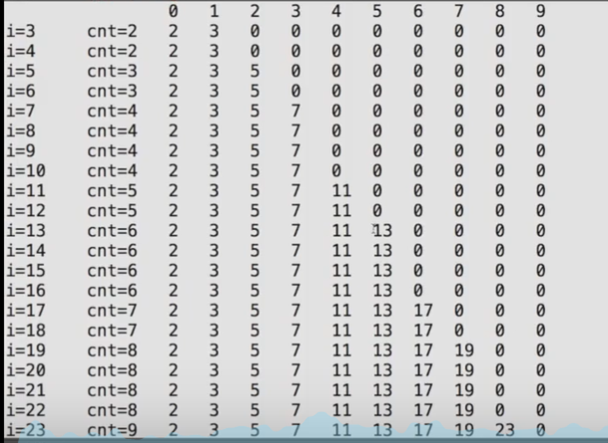1. 令x=2
2. 将2x,3x……直到ax<n的数标记为非素数
3. 令x为下一个没有被标记为非素数的数，重复2；直到所有的数都已经被尝试完毕。

（从2,3,4,5,6,7,8……删掉2的所有倍数，再删掉3的所有倍数，再删掉5的所有倍数……）

1. 先开辟数组prime[n]，初始化其所有元素为1，prime[x]=1表示x是素数，prime[x]=0表示x不是素数
2. 令x=2
3. 如果x是素数，则for(int i=2;x*i<n;i++)prime[i*x]=0
4. x++，重复3直到x==n，否则结束。

int main()
{
const int maxNumber=25;
int isPrime[maxNumber];
int i,x;
for(i=0;i<maxNumber;i++)
{
isPrime[i]=1;
}
for(x=2;x<maxNumber;i++)
{
if(isPrime[x])
{
for(i=2;i*x<maxNumber;i++) isPrime[i*x]=0;
}
}
printf("\n");
return 0;
}

## 二维数组

int a;通常理解为a是一个3行5列的矩阵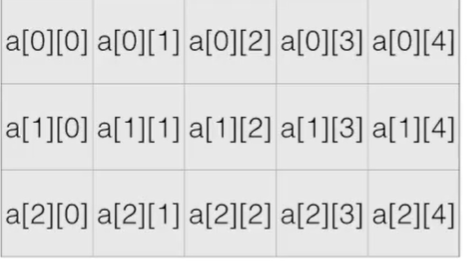int a[]={
{0,1,2,3,4},
{2,3,4,5,6},
};

a[i][j]表示一个int
a[i,j]是逗号运算符（等于逗号右边的值），表示a[j]，不是正确表达二维数组的方式。

PS: tic-tac-toe 井字棋判断输赢问题：行列对角线分开检查。

const int size = 3;
int board[size][size];
int i,j;
int num0fX;//X是一方
int num0fO;//O是一方
int result=-1;//-1：平局，1：X方赢，0:O方赢

//读入矩阵
for(i=0;i<size;i++){
for(j=0;j<size;j++){
scanf("%d",&board[i][j]);
}
}

//检查行
for(i=0;i<size&&result==-1;i++){
num0fO=num0fX=0;
for(j=0;j<size;j++){
if(board[i][j]==1)num0fX++;
else num0fO++;
}
if(num0fO==size)result=0;//O方赢
else if(num0fX==size)result=1;//X方赢
}

# 9. 指针

## 取地址运算：&运算符取得变量的地址

scanf里一定要加&（运算符）作用：取得变量的地址，其操作数必须是变量
C语言的变量是放在内存里的，&是取出该变量的地址

printf("0x%x",&i);//%x是以16进制输出；&i是一个地址

printf("%p",&i);

p=(int)&i;
printf("0x%x",p);

%p：会把值作为地址以十六进制输出，前面加0x

&不能对没有地址的东西取地址（&(a+b)&(a++)&(++a)）；&右边必须有一个明确的变量，才能去取这个地址。

int a;
int b;

## 指针 就是记录地址的变量

scanf的原型就是个函数，他一定有办法可以接收到该地址。（就是这个&）

*是一个单目运算符，用来访问指针的值所表示的地址上的变量。*可以做右值也可以做左值。

int i;
int *p=&i;//（p指向了i）*p理解为指向p指针地址所存在的值=i的地址。
int* p,q;
int  *p,q;

void f(int*p);
int main()
{
int i=6;
printf("&i=%p\n",&i);
f(&i);
return 0;
}
void f(int *p)
{
printf("p=%p\n",p);
}//该函数在被调用的时候得到了某个变量的地址

void f(int *p);

p是地址，*p是该地址中的数。

//我们调用的时候可以这么写：
int i=0;f(&i);

*&yptr->*(&yptr)->*(yptr的地址)->得到那个地址上的变量，即yptr
&*yptr->&(*yptr)->&(y)->得到y的地址，即yptr

## 指针的用处

1. 现在在函数里利用指针就能使交换两个变量成为可能。可以直接对该地址的变量改变值。和之前的swap函数（传递的是两个值）不一样。

void swap(int *pa,int *pb)
{
int t=*pa;
*pa=*pb;
*pb=t;
}

写一个求最大值最小值的函数，要返回最大值和最小值两个值。
int a,min,max;
minmax(a,sizeof(a)/sizeof(a),&min,&max);

void minmax(int a[],int len,int *min,int *max)
/*
sizeof(a)/sizeof(a)是数组元素个数。

1. 函数要返回运算的状态，结果通过指针返回。

给你两个数做除法，当分母等于0时返回0，分母不等于0的时候要返回1和除法的结果。
int divide(int a,int b,int *result)
{
int ret=1;
if(b==0)ret=0;
else{
*result=a/b;
}
return ret;
}

## 指针与数组：为什么数组函数之后的sizeof不对了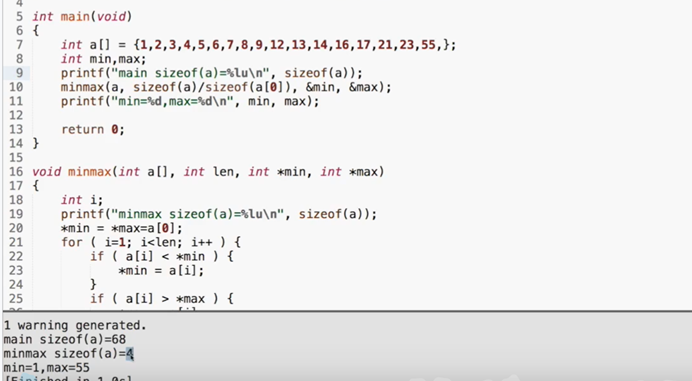sizeof(a)（a是个数组）返回的是int*的sizeof，而不是int []的sizeof

int sum(int *ar,int n);
int sum(int *,int);
int sun(int ar[],int n);
int sum(int [],int);

a==&a
[]运算符可以对数组做，也可以对指针做
P就相当于*P

pritnf("min=%d,max=%d\n",min,max);
int *p=&min;
printf("*p=%d\n",*p);
printf("p=%d\n",p);//得到的是一样的结果。

*a=a

int b[]->int * const b;

## 指针与const：指针本身和所指的变量都可能const（本节只适用于c99）

int * const q=&I;//q是const
*q=26//可以改变i的值
q++//error

const int *p=&I;
*p=26;//error!(*p)是const，不能通过p做赋值。
I=26;//可以直接改i
P=&j;//改变p指向的地址，可以

int I;
const int*p1=&I;
int const*p2=&I;
int *const p3=&I;

void f(const int*x);
int a=15;
f(&a);//可以
const int b=a;
f(&b);//可以
b=a+1;//不行！

Const int a[]={1,2,3,4,5,6};

int sum(const int a[],int length);

## 指针运算

char a[]={0,1,2,3,4,5,6}
char *p=ac;
printf("p的地址是%p\n",p);
printf("p+1的地址是%p\n",p+1);

0xbffbbd5e
0xbffbbd5f

*p++：取出p所指的数据，之后顺便把指针移动到下一个位置。（++的优先级比*高）不需要括号，常常用于数组类连续空间。

p=ac;
while(*p!=-1)
{
printf("%d\n",*p++);
}

（在某些CPU上，*p++被直接翻译成一条指令，使得取得p地址内的值之后，p指针++。）
>,>=,<,<=,!=也都可以对指针做，比较指针在内存中的地址。数组中单元的地址是线性递增的。

0地址

1.返回的指针是无效的
2.指针没有真正被初始化（先初始化为0地址）
NULL是一个预定定义的符号，表示0地址，建议使用，因为有的编译器不接受用0来表示0地址。

void*表示不知道指向什么东西的指针，计算时与char*相同。

int *p=&i;void*q=(void*)p;

## 动态内存分配

C99可以用变量做数组定义的大小。C99之前该怎么做？

int *a=(int*)malloc(n*sizeof(int));

#include<stdlib.h>
{
int n;
int *a;
scanf("%d",&n);
a=(int*)malloc(n*sizeof(int));
//用完之后
free a;
}

free()：把申请的空间还给系统，只能还该空间的首地址。一定要还。

void *p=0;之后再free(p)，不管有没有使用malloc，free()都没有问题。

# 10. 字符串

char word[]={'H','e','l','l','o','!'};

wordH
worde
wordl
wordl
wordo
word!

0和'\0'是一样的，但是和'0'不同。0标志着字符串的结束，但他自己不是字符串的一部分，计算字符串长度的时候不包含这个0。

PS:string.h里有很多处理字符串的函数。
char *str="Hello";//一个指针指向了一个字符数组
char word[]="Hello";//字符数组，结尾0
char line="Hello";//字符数组，结尾0

"Hello"双引号括起来的叫做字符串的字面量/常量，我们在scanf和printf里已经见过很多次了。"Hello"会被编译器变成一个（长度为6的）字符数组放在某处，结尾自动添上个0。

printf("123456789"
"10111213");

printf("123456789\
10111213");

C语言的字符串是以字符数组的形态存在的，因为是个数组，所以不能用运算符对字符串做运算。但可以通过数组的方式遍历字符串。（后面会有很多应用）

## 字符串变量

char *s="Hello";
s='B';//尝试把H替换为B，然后输出s

int i=0;
char *s="Hello";
char*s1="Hello";//两个一样的字符串变量
printf("i的地址是%p\n",&i);
printf("s的地址是%p\n",s);
printf("s1的地址是%p\n",s1);

1.i的地址相对很大，s和s1的地址相对很小。
2.s和s1的指向了同一个地址。

char s[]="Hello!";

1. 字符串有确定的地址；
2. 作为本地变量，空间会自动被回收。

1. 只读的，不可写的字符串
2. 处理函数的参数（前面知道如果数组作为指针，函数的参数实际上和指针是一样的）；
3. 动态分配空间malloc。

## 字符串输入输出

char *t="title";
char *s;
s=t;

char string;
scanf("%s",string);
printf("%s",string);

scanf()只能读入一个单词，因为碰到空格、tab键或回车就会终止。空格、回车是分隔符，是用来分隔两个单词的。

scanf("%s",str1);
scanf("%s",str2);
printf("%s%s",str1,str2);

scanf()是不安全的，因为不知道读入的长度。

char str1,str2;
scanf("%s",str1);
scanf("%s",str2);

C语言中常见的错误：误以为char*就是定义了字符串类型。其实是一个需要初始化的指针。不初始化可能会出错。（可能会出现：程序在一个地方没问题，到了其他地方就出错了）

char zero="";

## 字符串数组，以及程序参数

char **a：a是个指针，指向另一个指针，另一个指针指向一个字符串
char a[][]：a是个二维数组的变量，第二维（后面的括号）一定要有确定的大小，否则编译不能通过。

char a[]={
"hello",
"world"
};//每个字符串长度不要超过n，即10

argc告诉我们，后面的数组有多少个字符串。

for(i=0;i<argc;i++){
printf("%d:%s\n",i,argv[i]);
}

0:./a.out
1:123

## 字符、字符串操作

### 单字符输入输出

putchar(int c)函数：向标准输出写一个字符，但是输入是int类型，返回类型也是int 表示写了几个字符，一般为1，EOF(即：值-1)表示失败。(end of fail)
getchar()：从标准输入读入一个字符，返回类型也是int(因为要返回EOF表示输入结束了）

int ch;
while((ch=getchar())!=EOF){
putchar(ch);
}
printf("EOF\n");//这样来看读入什么会EOF
return 0;

shell对输入的东西做了行编辑，也就是敲下回车前输入的部分都在shell里处理，放在缓冲区里，按下回车后才送到程序那里。如：输入123，shell缓冲区里为“1，2，3，回车”。然后getchar()再读缓冲区。

### 字符串函数strlen

string.h头文件中处理字符串的函数比如：strlenstrcmpstrcpystrcatstrchrstrstr
strlen(const char*s)：返回s的字符串长度（不包括结尾0）由参数表中的const可知该函数不会修改传入的数组。

char line[]="Hello";
printf("%lu\n%lu",strlen(line),sizeof(line));

sizeof不行，因为我们得到的是指针所占据的大小。

int cnt=0;
while(s[cnt]!='\0'){
cnt++;
}
return cnt;

### 字符串数组strcmp

int strcmp(const char*s1,const char*s2)：比较两个字符串。

0相等
1s1大
-1s2大

printf("%d\n",s1==s2);来判断s1和s2是否相等。

s1[]="abc";
s2[]="bbc";

s1[]="abc";
s2[]="Abc";

s1[]="abc";
s2[]="abc ";//多了个空格

while(s1[idx]!='\0'&&s1[idx]==s2[idx])//当出现不相等的字符或者字符串到了末尾时，返回差值
idx++;
return s1[idx]-s2[idx];

*s1==*s2
s1++;
s2++;
return *s1-*s2;

### 字符串函数strcpy

char*strcpy(char* restrict dst,char* restrict src);

restrict表示src和dst不能重叠。比如src是
H E L L O \0
，dst的内容是:
空 空 空 H E L L O \0

char *dst=(char*)malloc(strlen(src)+1);//不包含结尾的\0，所以+1
strcpy (dst,src);

+1是重点。

char *mycpy(char*dst,const char*src)
{
int idx=0;
while(src[idx]!='\0')
{
dst[idx]=src[idx];
idx++;
}
dst[idx+1]='\0';
return dst;

char *ret=dst;
while(*src!='\0')
{
*dst=*src;
*dst++;
*src++;
}
*dst='\0';
return ret;

while(*src)*dst++ = *src++;

while(*dst++ = *src++);

### 字符串函数strcat

char*strcat(char* restrict s1,const char* restrict s2);

   s2:W O R L D\0

s1必须要有足够的空间。
cpy和cat有可能没有足够的空间，因此这两个函数是不够安全的，不建议使用。

char*strncpy(char* restrict s1,const char* restrict s2,size_t n);
char*strncat(char* restrict s1,const char* restrict s2,size_t n);
char*strncat(const char* s1,const char* s2,size_t n);

### 字符串搜索函数

char* strchr(const char* s,int c);
char* strrchr(const char* s,int c);//从右边开始找

char *s="hello";
char *p=strchr(s,'l');
printf("%s",p);

p=strchr(p+1,'l');

char *t=(char*)malloc(strlen(p)+1);
strcpy(t,p);
free(t);

char c=*p;
*p='\0';//把l的位置改成'\0'
char *t=(char*)malloc(strlen(s)+1);
strcpy(t,s);//只拷贝了第一个l前面的部分。

t就是想要的结果，即he

# 11. 枚举

const int red=0;
const int yellow=1;
const int green=2;

int main()
{
int color=-1;
char *colorname=NULL;
printf("请输入你喜欢的颜色的代码");
scanf("%d",&color);
switch(color){
case red:colorname="red";break;
case yellow:colorname="yellow";break;
case green:colorname="green";break;
default:colorname="unknown";break;
}
printf("%s",colorname);
return 0;

enum COLOR{RED,YELLOW,GREEN};
int main(){
int color=-1;
char *colorname=NULL;
printf("输入你喜欢的颜色代码：");
scanf("%d",&color);
switch(color){
case RED:colorname="red";break;//在case处就可以直接使用RED YELLOW和GREEN来取代0,1,2
case YELLOW:colorname="yellow";break;
case GREEN:colorname="green";break;
default:colorname="unknown";break;
}
printf("你喜欢的颜色是%s\n",colorname);
return 0;
}

enum 枚举类型名{名字0,名字1……名字n};enum是enumeration。

enum color {red,yellow,green};
void f(enum color c);
int main()
{
enum color t= red;
scanf("%d",&t);
f(t);
return 0;
}

void f(enum color c)
{
printf("%d\n",c);
}

enum COLOR{RED,YELLOW,GREEN,numcolors};//结尾的numcolors表示数组的结尾，同时也可以表示enum中元素的个数。
int main()
{
int color=-1;
char *ColorNames[numcolors]={
"red","yellow","green",
};
char *colorname=NULL;
printf("请输入你喜欢的颜色的代码");
scanf("%d",&color);
if(color>=0&&color<numcolors) colorname=ColorNames[color];
else colorname="unknown";
printf("你喜欢的颜色是%s",colorname);
return 0;
}

enum color{red=1,yellow,green=5};如果输出%d,green 就会输出5。

## 结构类型

int main()
{
struct date{
int day;
int month;
int year;
};//声明时，结尾有个分号！！
struct date today;//像枚举一样不要忘记开头，struct
today.day=12;
today.month=3;
today.year=2021;
printf("Today's date is %i-%i-%i.",today.year,today.month,today.day);
return 0;
}

struct{
int x;
int y;
}p1,p2;

p1和p2都是一种无名结构，都包含x和y。没有声明结构名字，临时造了两个无名结构出来。

struct point{
int x;
int y;
}p1,p2;

p1,p2都是point，都包含x和y。

monthdayyear
11232007
today的内存中包含month, day, year.

struct date={12,3,2021};//注意顺序
struct thismonth={.month=3,.year=2021};//剩下没有被赋值的部分都是0，和数组一样

p1=(struct point){5,10};
p1=p2;

struct date today,day;
today=(struct date){12,3,2021};
day=today;

struct date *pdate=&today;

## 结构与函数

int numberofdays(struct date d)

（貌似美国的写法是月/日/年）
&date.month中，取成员运算符.的优先级高于取地址运算符&

struct point p={0,0};
gtestruct(p);

void getstruct(struct point p){
scanf("%d",&p.x);
scanf("%d",&p.y);
}

struct point getstruct(void)
{
struct point p;
scanf("%d",&p.x);
scanf("%d",&p.y);
return p;
}
//main函数中：y=getstruct();

struct date myday;
struct date *p=&myday;
(*p).month=12;//正常应该这样写
p->month=12;//也可以简写成这样

->表示指向结构变量中的成员

#include<stdio.h>
struct point{
int x;
int y;
}p;

struct point *getstruct(struct point *p);
void print(const struct point *p);

int main()
{
struct point p={0,0};
print(getstruct(&p));
return 0;
}

struct point *getstruct(struct point *p)
{
scanf("%d",&p->x);
scanf("%d",&p->y);
return p;
}//像这样传入一个参数，对其做处理后再返回该参数的函数，可以直接套用在其他函数中。

void print(const struct point *p)//const
{
printf("%d %d",p->x,p->y);
}

## 结构中的结构

struct date dates;
struct date dates[]={
{3,21,2021},{3,22,2021}
};
printf("%.2i",dates.month);//不知道%.2i什么意思

struct point{
int x;
int y;
}
struct rectangle{
struct point pt1;
struct point pt2;
}
//如果有这样的定义：
struct rectangle r;
/*则可以有：
r.pt1.x;
r.pt1.y;
r.pt2.x;
r.pt2.y;
*/
//如果有这样的定义：
struct rectangle *rp;
rp=&r;
//那么下面的四种形式是等价的
r.pt1.x
rp->pt1.x
(r.pt1).x
(r->pt1).x
//但是不能写r->pt1->x,因为pt1不是指针而是结构。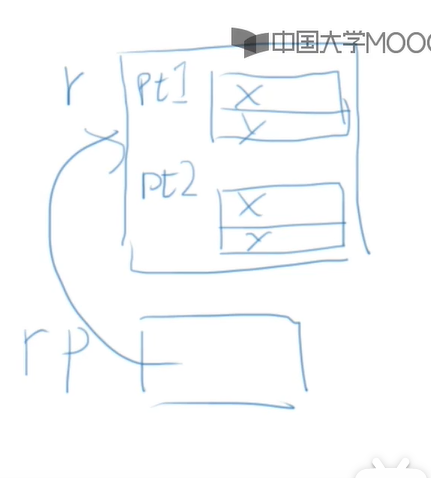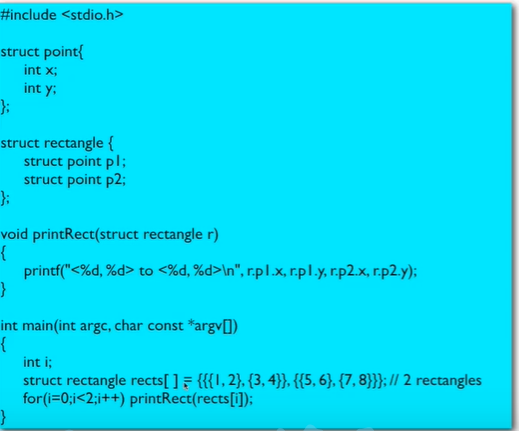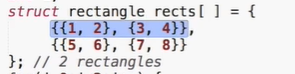（这里也能看出这么写会好看很多~）

## 类型定义

typedef int length;

length a,b;
length a;

typedef struct ADate{
int month;
int day;
int year;
}Date;

Date a={3,24,2021};
typedef *char String;//string是十个字符串的数组的类型。

## 联合

union，表面上与struct非常相似。

unit xxx{
int a;
char b;
}xxx1,xxx2;

xxx1.a=1;
xxx2.b='c';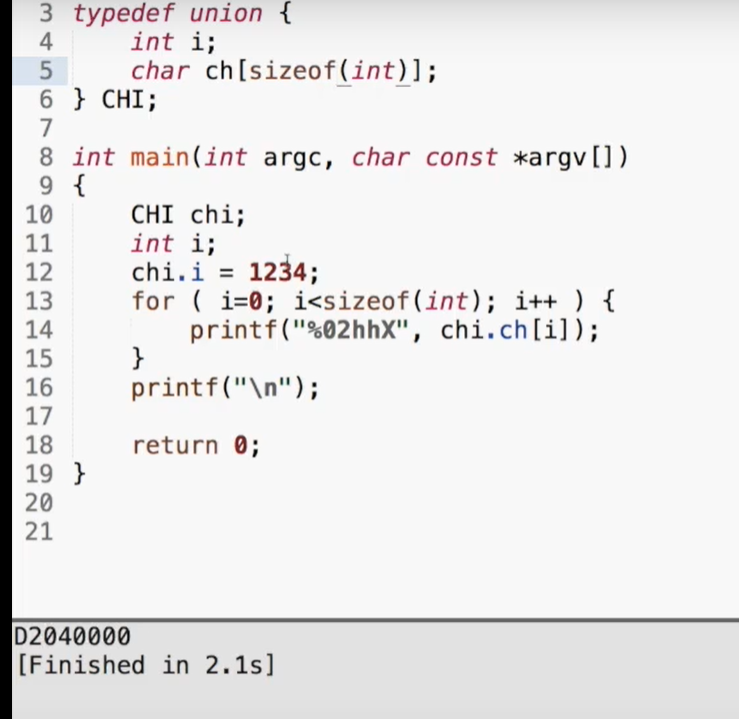2.这就是一个字节了，不要再扩展了
3.以十六进制输出

# 12. 全局变量

## 全局变量：定义在函数之外的变量，全局的生存期和作用域

int gAll=12;
int main()
{
printf("in %s, gAll is %d",__func__,gAll);//__func__是输出当前函数名称
return 0;
}

## 静态本地变量：能在函数结束后继续保持原值的本地变量

f(){
static int a=1;
a+=2;
}

f()连续执行三次，不是每次都会a=1，而是1 3 3 5 5 7。

## 后记：返回指针的函数，使用全局变量的贴士

int *f();
void g();

int main()
{
int *p=f();
printf("*p=%d",*p);
g();
printf("*p=%d",*p);
return 0;
}
int *f()
{
int i=12;
return &i;
}
void g()
{
int k=24;
printf("k=%d",k);
return 0;
}

tips
1.不要用全局变量在函数之间传递参数和结果（可以，但是有问题。详见丰田汽车案？？）。使用全局变量和静态本地变量的函数是线程不安全的，尽量避免全局变量。

## 宏定义

#define PI 3.14159

gcc：

gcc xxx.c --save-temps
ls-l

tail xxx.c;
tail xxx.i;

# define会先对程序进行预处理，把宏都替换掉；

#define 单词 值

# define会对后面的值原封不动地进行文本替换，所以千万小心结尾不要加分号。因为这不是c的语句，c的语句结尾才需要加分号。

#define prt printf("123");\
printf("456");

c编译器里有一些预先定义的宏，可以直接用的

__LINE__//行号
__FILE__//文件名
__DATE__//编译时日期
__TIME__//编译时时间
__STDC__

## 带参数的宏

#define cube (x)((x)*(x)*(x))

printf("%d\n",cube(5));//输入tail 文件名，输出((5)*(5)*(5))

RADTODEG(x) (x*57.29578);
RADTODEG(x) ((x)*57.29578);

#define MIN(a,b) ((a)>(b)? (b):(a))

C语言有inline机制，有类型检查，也许会逐渐取代宏。

# 13. 文件和位运算

## 多个源代码文件

main中的代码太长，可以分出几个函数；而一个源代码文件(.c)太长可以分出很多.c文件。

## 头文件

int max(int a,int b);如果不加这条声明，其实C语言也会默认a和b是Int类型的。

main中默认a,b是int→传入double 的max→以int形式传回来，链接的确没有问题，但传入传出都是错的。

1.再新建一个源代码文件，会提示要不要在项目中添加，点yes；
2.命名为.h的文件（如max.h）输入一句话，就是那句函数的原型
int max(int a,int b);
3.在所有调用这个函数的源代码文件：main.c和max.c文件中都加入该头文件

#include"max.h"

# include是一个编译预处理指令，会把该头文件下的所有内容原封不动地插入他所在的地方；因此也不是必须在最开头。

""：先去找系统内指定目录有没有这个头文件，没有再去当前目录找。
<>：只在系统内找这个头文件。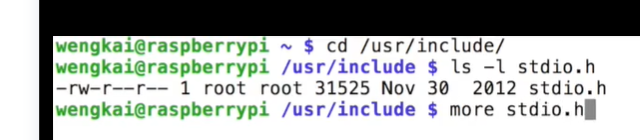stdio.h里只有printf的原型，其代码在另外的地方。比如windows在.lib里，unix在.a里。而stdlib.h里有malloc的原型

# include<stdio.h>只是为了让编译器知道printf函数的原型，保证调用时给出的参数值是正确的类型。

## 声明

extern int gAll;
int x;是变量的定义；extern int x;是变量的声明。

#ifndef __MAX_H__
#define __MAX_H__//如果没有定义过max.h则定义max.h

//中间是声明

#endif

# pragma once也能起到相同的作用，但是并不是所有的编译器都支持。所以还是用标准头文件结构。

## 格式化输入输出

printf: %[flags][width][.prec][hIL]type
flag：标志，有以下几种

flag含义
-左对齐（和width一起用）
+输出前面带正负号
(space)正数留空
0空格用0填充（不能和-同时使用）
width（宽度）或prec
width或prec含义
number最小字符数（包括小数点）
*下一个读入的参数是字符数
.number小数点后面的位数
.*下一个参数是小数点后的位数
hIL：类型修饰符

hh单个字节
hshort
llong
lllong long
Llong double
type：类型
type用于
i或dint
uunsigned int
o八进制
x十六进制
X大写字母的十六进制
f或Ffloat, 6
e或E指数
gfloat
Gfloat
a或A十六进制浮点
cchar
s字符串
p指针
n读入/写出的个数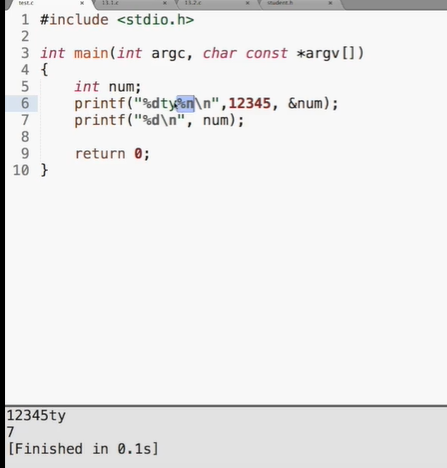%n是当操作做到这里时，已经输出了多少个字符，并且填到指针所指的变量里。
scanf: %[flag]type
flag含义
*跳过

hhchar
hshort
llong, double
lllong long
Llong double
type用于
dint
i整数，可能是十六进制或八进制
uunsigned int
o八进制
x十六进制
a, e, f, gfloat
cchar
s字符串（单词）
[...]所允许的字符
p指针
%i会根据输入（如0x12、012）来判断是十六进制还是八进制还是十进制。
[...]举例
GPS中会读到一串字符，用逗号分隔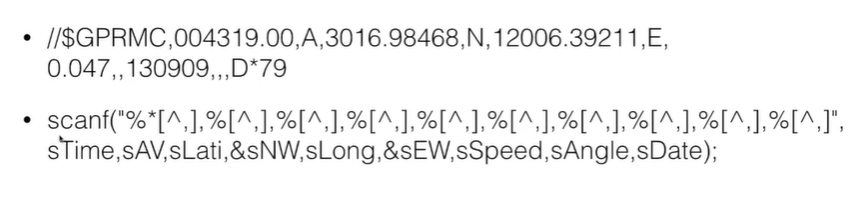printf和scanf是有返回值的。scanf是返回几个item，即这次读入了几个变量；而printf则是这次输出了多少个字符。因此当我们面对要求严格的程序，比如长期运行的大程序，就需要判断每次调用scanf和printf的返回值来了解程序运行中是否会存在问题。

## 文件输入输出

（当然还可以./test < 12.in > 12.out，输入是文件，输出也是文件。12.out里也有我们所期望的结果。）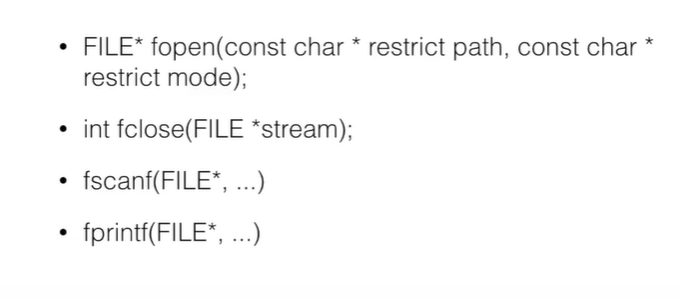FILE* fp=fopen("file","r");//file是文件名，r表示读
if(fp){//如果没打开，会返回NULL
fscanf(fp,...);//读文件。省略号的东西和正常的scanf一样了。后面还可以printf
fclose(fp);
}else{}//无法打开的反馈。如：输出 无法打开文件

fopen第一个参数是文件名的字符串，第二个参数字符串用途如下：

r打开只读
r+打开读写，从文件头开始读/写
w打开只写，如果不存在则新建，如果存在则清空
w+打开读写，如果不存在则新建，如果存在则清空
a打开追加，如果不存在则新建，如果存在不清空，从文件尾开始

## 二进制文件

UNIX（和windows一样都是操作系统。）喜欢用文本文件储存数据、配置程序。交互式终端的出现（类似windows的cmd窗口）使得人们喜欢用文本和计算机“交流”。因此，UNIX的shell就提供了一些读写文本的小程序。
windows（更准确要说DOS），个人计算机的制作者并不继承、熟悉UNIX文化（宛如在围墙之外，不熟悉围墙内）全凭自己的理解做出草根文化的DOS，更喜欢用二进制文件。
PC和DOS刚开始的时候能力有限，二进制更加接近底层。

1. 有配置（比如窗口大小、字体颜色）UNIX用文本文件就能编辑，Windows是用一个大文件：注册表编辑。
2. 数据：保存数据，如学生成绩。稍微大一些的数据都放数据库那了。
3. 媒体：如图片音频，这些不能是文本文件，只能是二进制文件。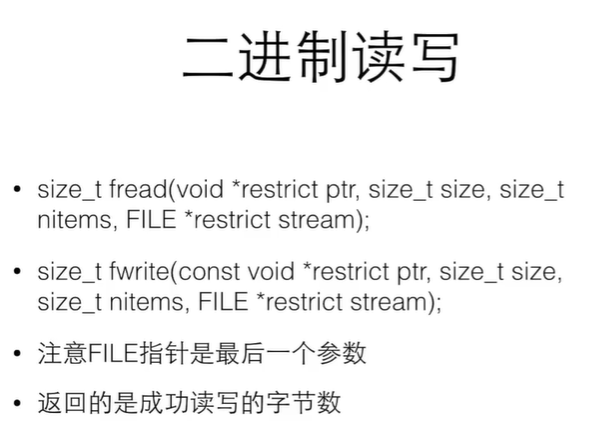long ftell(FILE *stream);
int fseek(FILE *stream, long offset, int whence);
/*whence：SEEK_SET 从头开始
SEEK_CUR 从当前位置开始
SEEK_END 从末尾开始*/

## 按位运算

·&按位的与
·\ 按位的或
·~按位取反
·<<左移
·>>右移

0101 1010 5A
1000 1100 8C 做运算后：
0000 1000 08

1. 让某一位或某些位=0，如x & 0xFE（1111 1110） 会让最后一位变为0
2. 取某个数中的某一段：如x & 0xFF (在32位int中是 0000 0000 0000 0000 0000 0000 1111 1111）这样只会留下最后8位

1. 使得某一位/某几位变为1： x | 0x01
2. 把两个数拼起来： 0x00FF | 0xFF00

## 移位运算

i<<j：i中所有位向左移动j个位置，右边填入0

## 位运算例子

unsigned后面没有跟类型，则是默认为unsigned int。
1000 0000
0100 0000
0010 0000
...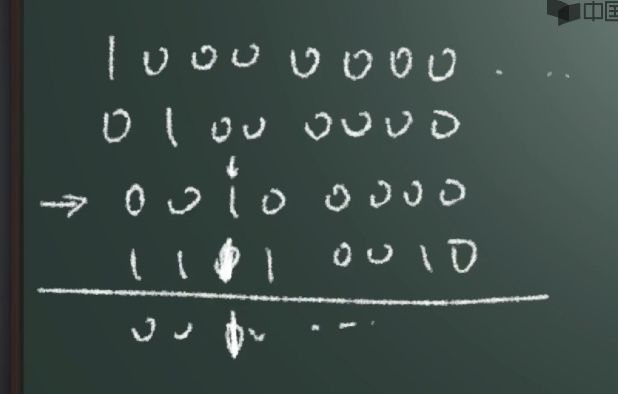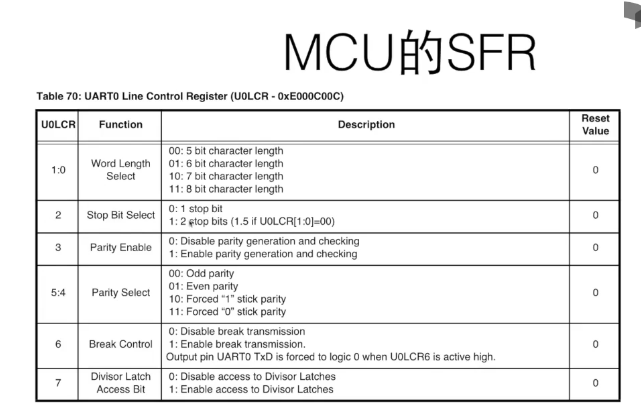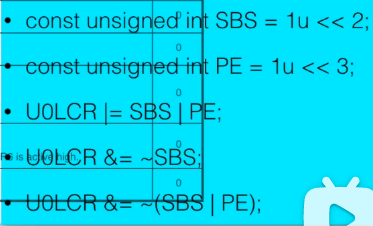PE是1，左移3位是1000
100
1000
1100（或后得到的结果）

## 位段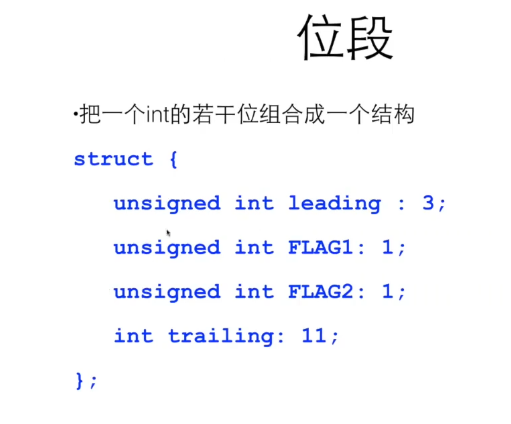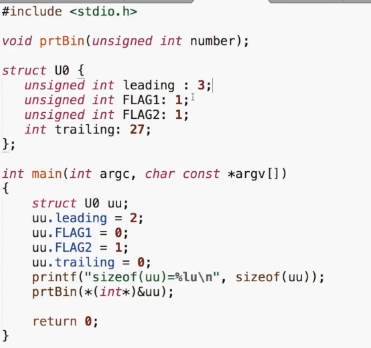prtBin函数就是刚刚看过的输出二进制位。

# 14. 可变数组

resizable array
growable 可变大的
get the current size 能知道现在的大小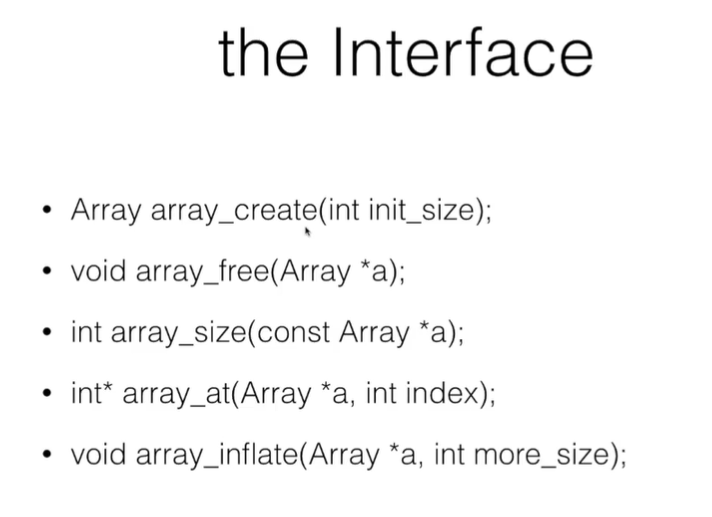create：创建数组
free：回收数组空间
size：告知单元数量
at：访问某个单元
inflate：长大

Array array_create(int init_size){
Array a;
a.size=init_size;
a.array=(int*)malloc(sizeof(int)*init_size);
return a;
}

void array_free(Array *a)
{
free(a->array);
//保险起见，令a->size=0, a->array=NULL
}

## 可变数组的数据访问

size：读入结构指针，直接return a->size即可。

at

int *array_at(Array *a, int index)
{
return &(a->array[index]);
}

void array_get(const Array *a,int index)
{
return a->array[index];
}
void array_set(Array *a,int index,int value)
{
a->array[index]=value;
}


## 可变数组自动增长

void array_inflate(Array *a, int more_size)
{
int *p=(int*)malloc(sizeof(int)*(a->size+more_size);
int i;
for(i=0;i<a->size;i++)p[i]==a->array[i];//把老空间里的东西放到新空间里去。
free(a->array);
a->array=p;
a->size+=more_size;
}

block概念：每次多申请block，const block_size=常数，
array_inflate(a,(index/block_size+1)*block_size-a->size);多申请这些空间。

while(number!=-1)
{
scanf("%d",&number);
*array_at(&a,cnt++)=number;
}

## 可变数组的缺陷

1. 每次都要拷贝，很花时间
2. 每次都是在之前的内存结尾申请空间，不断往后排，所以最后其实我们不需要那么多内存空间，但我们的内存空间不够了。
3. 如果我们每次都只申请一块block大的内存，把他们链起来，不需要拷贝了节约时间，也可以充分利用给内存的每一块。

# 15. 链表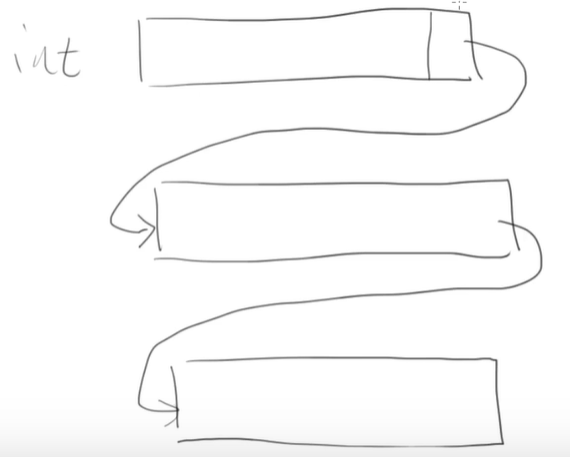typedef struct _node{
int value;
struct _node *next;
}Node;

do{
scanf("%d",&number);
}while(number!=-1);

Node *head=NULL;
Node *p=(Node*)malloc(sizeof(Node));
p->value=number;
p->next=NULL;
//find the last, attach
{
while(last->next)last=last->next;
last->next=p;


## 链表的函数

void add(Node *head,int number);

last=head;
while(last->next)last=last->next;//找到结尾last结点
last->next=p;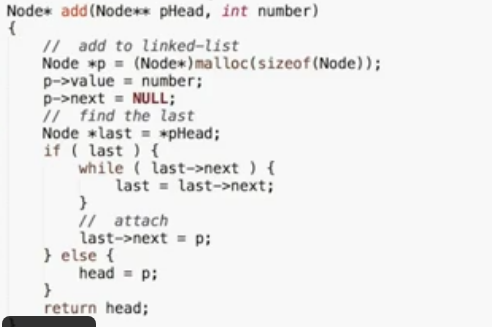## 链表的搜索

node *p;
for(p=list.head;p;p=p->next)printf("%d\t",p->value);//遍历

for(p=list.head;p;p=p->next)
{
if(p->value==number)printf("找到了！");
}

## 链表的删除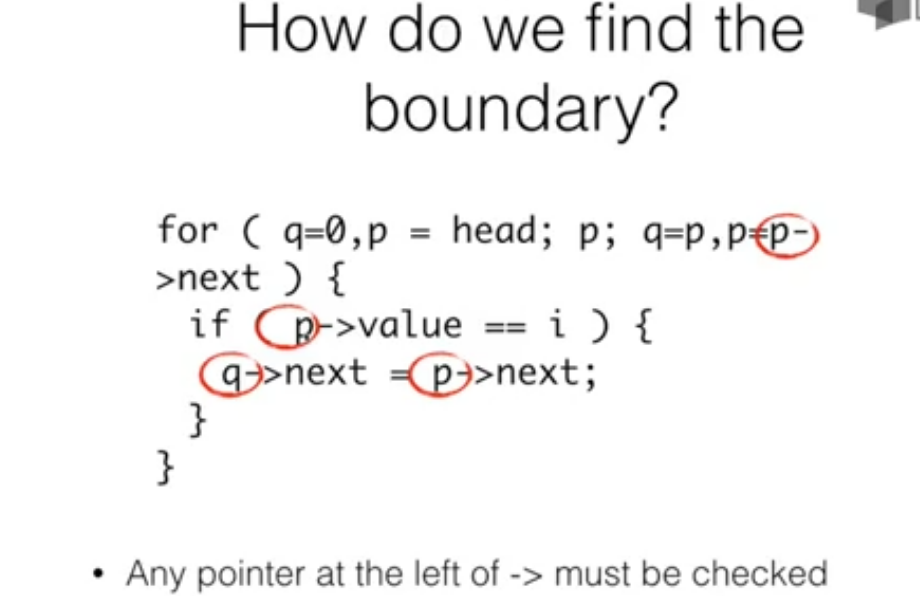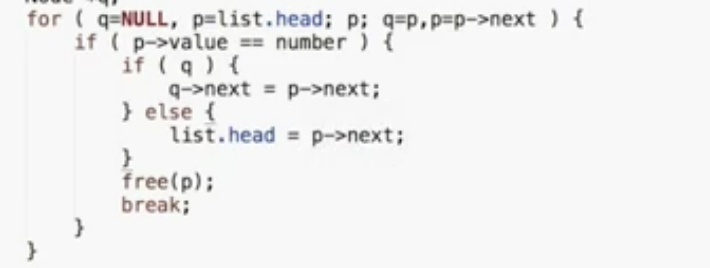## 链表的清除

for(p=head;p;p=q)
{
q=p->next;
free(p);
}

# 完结|
10天前
|

C语言（一）数据类型笔记 梦开始的地方
C语言（一）数据类型笔记 梦开始的地方
10 0
|
2月前
|

41 0
|
2月前
|

C语言笔记第03章：数组（四）
C语言笔记第03章：数组
21 0
|
2月前
|

C语言笔记第03章：数组（三）
C语言笔记第03章：数组
34 0
|
2月前
|
C语言 索引
C语言笔记第03章：数组（二）
C语言笔记第03章：数组
73 0
|
2月前
|

C语言笔记第03章：数组（一）
C语言笔记第03章：数组
28 0
|
2月前
|

C语言笔记第02章：三大基本结构（三）
C语言笔记第02章：三大基本结构
15 1
|
2月前
|
Linux C语言
C语言笔记第02章：三大基本结构（二）
C语言笔记第02章：三大基本结构
19 0
|
2月前
|

C语言笔记第02章：三大基本结构（一）
C语言笔记第02章：三大基本结构
26 0
|
2月前
|
Java 程序员 编译器
C语言笔记第01章：初识C语言（二）
C语言笔记第01章：初识C语言
40 0